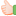# Thread: Lambert W function for Excel, work on real and complex number

1. ##Lambert W function for Excel, work on real and complex number

The Lambert W function (very efficient, fast)

See, LambertW.bas to get the VB module (attach)

Input: real or complex values from -infinity to +infinity
You can verify the results with

=IMSUM(IMPRODUCT(LambertW(COMPLEX(-100,0)),IMEXP(LambertWc(-100,0))),-1)
return:
-100.999999999999+8.5265128291212E-14i

OR

=IMPRODUCT(LambertW(-0.1),IMEXP(LambertW(-0.1)))
return:
-0.1

Lots of testing, no error foundReply With Quote

2. ## Re: Lambert W function for Excel, work on real and complex number

Thank you! That has saved me a lot of work!Martin/Reply With Quote

3. ## Re: Lambert W function for Excel, work on real and complex number

Thank you also.

One minor quibble: there seems to be an issue with the results that come from input values between 0 and -0.0212. As soon as it hits -0.0212 it's like hitting a wall, beyond that the results are wildly off:

-0.0218 > -0.02229
-0.0217 > -0.02219
-0.0216 > -0.02208
-0.0215 > -0.02198
-0.0214 > -0.02187
-0.0213 > -0.02177
-0.0212 > -5.51714
-0.0211 > -5.57716
...and so on, all input values from there to zero are similarly off.

Can anyone see why? Unfortunately I just lack the coding skills to pick it apart - gave it a go, but just no experience there whatsoever :-(

Any help much appreciated.Reply With Quote

4. ## Re: Lambert W function for Excel, work on real and complex number

"If x is within the sub interval (-1/e, 0), the calculator returns two values."

And for x = -0.0211, the calculator returns -5.577162 and -0.02156, both values are valid.
In Excel, the function return only 1 of them, I will check if i can return the more suitable one.Reply With Quote

5. ## Re: Lambert W function for Excel, work on real and complex number

Aha, that all makes sense. Thank you.

Please don't spend any more time on my account - now the dual correct answers are apparent I can work with that.

Thanks again.Reply With Quote

6. ## Re: Lambert W function for Excel, work on real and complex number

I searched high and low for access top a vba routine for Lambert W.

This was the best - BUT it sometimes gives the -ve branch solutions instead of the +ve.

It is also complex which i do not need.

For others I will post my solution here.

It returns the +ve branch solution only, is accurate to 9dp for most of the useful space and is reasoanbly faast

Here it is and hope others find it useful.

Bob J.

Code:
```Public Function myLambertW(x As Double) As Double
'
'      Function provided on the web  seems to have starting problems and uses complex Nos where I only need real
'      This version solves for the upper branch of the solution only
'
'      It uses standard Newton iteration
'
'      The starting value is log(x+1) which is within 40% of target from -0.33 < x < 10^100
'
'      Results are accurate to about 9dp from -0.3678 < x < 10^100
'
'      Values for second Branch are not computed ie -0.36788... < x < 0, W(x) < -1
'
'      There is some potential to reduce iterations for smaller +ve x values
'      but may need to increase as x approaches -1
'
'                           RB Jordan 3/2/2015
'

Dim xTry As Double
Dim Iter As Integer

If x < -Exp(-1) Then
myLambert = CVErr(xlErrValue)
Exit Function
End If

xTry = Log(1 + x)
For Iter = 1 To 9
xTry = xTry - (xTry - x / Exp(xTry)) / (1 + xTry)
Next Iter

myLambertW = xTry

End Function```Reply With Quote

7. ## Re: Lambert W function for Excel, work on real and complex number

All:

There is a very nice presentation of the Lambert's W function (W0) and a method to calculate it based upon Halley's method in MATLAB at http://blogs.mathworks.com/cleve/201...e-1773193df571. (Google: The Lambert W Function Cleve Moler if link inactive).Reply With Quote

function, visual basic####Posting Permissions

• You may not post new threads
• You may not post replies
• You may not post attachments
• You may not edit your posts
•